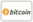Some Thermoelastic Problems on a Cylinder的封面

# Some Thermoelastic Problems on a Cylinder

LAP LAMBERT Academic Publishing (2016-10-21 )有获得代金券的资格
ISBN-13:

### 978-3-659-97446-5

ISBN-10:
3659974463
EAN:
9783659974465

This book contains mathematical aspects of direct and inverse thermoelastic problems on a cylinder. The concept of direct and inverse thermoelastic problems is the central theme of this book, finding its roots and applications in branches of mathematics, aeronautical sciences and mechanical engineering which is helpful for students and researchers. All the results are obtained in terms of Bessel's function in the form of infinite series. The numerical results are derived and depicted graphically. The mathematical approach of this book is to study new dimensions of thermoelastic problems in different areas.

https://www.lap-publishing.com/

504

2016-10-21

89.90 €

direct problems, Integral Transform, Inverse problem, Thermoelastic Problems### 时事通讯